# Consider the following linear program: Assuming no bounded-variable algorithm is available, solve by

Consider the following linear program: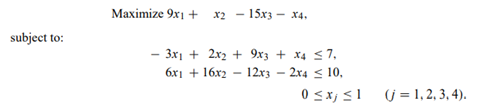Assuming no bounded-variable algorithm is available, solve by the decomposition procedure, using 0 ≤ x j ≤ 1 (j = 1, 2, 3, 4) as the subproblem constraints

. Initiate the algorithm with two proposals: the optimum solution to the subproblem and the proposal x1 = 1, x2 = x3 = x4 = 0.

Consider the following linear-programming problem with special structure: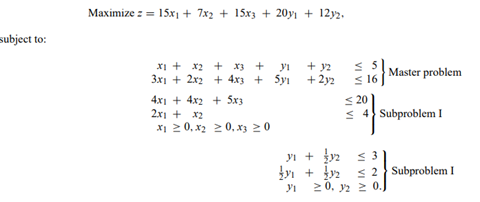Tableau 1 represents the solution of this problem by the decomposition algorithm in the midst of the calculations. The variables s1 and s2 are slack variables for the first two constraints of the master problem; the variables a1 and a2 are artificial variables for the weighting constraints of the master problem.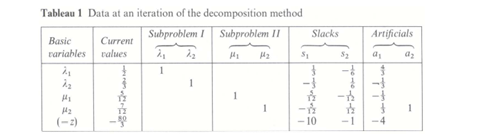The extreme points generated thus far are: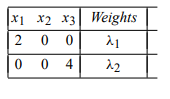for subproblem I and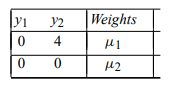for subproblem II

a) What are the shadow prices associated with each constraint of the restricted master?

b) Formulate the two subproblems that need to be solved at this stage using the shadow prices determined in part (a).

c) Solve each of the subproblems graphically.

d) Add any newly generated extreme points of the subproblems to the restricted master.

e) Solve the new restricted master by the simplex method continuing from the previous solution. (See Exercise 29 in Chapter 4.)

f) How do we know whether the current solution is optimal?